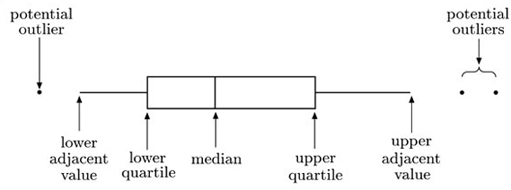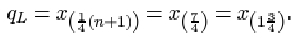Now that you’ve learned the principles of doing division on paper, you may want to practise your new skills. If so, go to the Dividing decimals page of the math.com website and follow the instructions. You will need a pen and paper to carry out each calculation. You can then enter your answer on the website to check if it is correct.

Author(s): The Open University

Doing division when decimal numbers are involved is the same as doing divisions involving whole numbers, with a few extra steps to take care of the decimal point.

Either the number you are dividing into or the number you are dividing by, or both of them, may be a whole number or a decimal number. So, for example, you might want to do the following divisions:

• Example 1: 49.26457 ÷ 8
• Example 2: 2.601 ÷ 1.22
• Example 3: 678 ÷ 27.356
Author(s): The Open University

In the previous sections you saw how to divide a big number by a small number up to 10. Things get harder if you want to do a division where both the numbers are big. This kind of calculation is called long division, probably because you write the steps of the calculation out on paper in a long sequence.

The principle of doing long division is the same as when you divide by a number up to 10. The only difference is that, because the numbers involved in long division are usually too big
Author(s): The Open University

A decimal number is a different way of representing numbers smaller than one. You put them after a full stop (the decimal point), for instance 0.5. The first digit after the decimal point represents tenths. If you sliced a cake into 10 slices, each slice would be a tenth of the cake. So 0.5 is the same as saying 5 tenths, and can be written [Image_Link]https://www.open.edu/openlearn/ocw/pluginfile.php/89018/mod_oucontent/oucontent/739/9849fb8c/1931b6c5/div_a
Author(s):
The Open University

An important thing to remember about division is that it has different rules from multiplication.

For example when you multiply two numbers together, it doesn’t matter what order you multiply them in. So 8 x 4 is exactly the same as 4 x 8. The result is 32 in both cases.

But in division, order matters. So 8 ÷ 4 is different from 4 ÷ 8.

The answer to the first is 2. If you multiply 4 by 2 you get 8.

But the answer to the second is 0.5 (a half). If you multiply 8 by
Author(s): The Open University

Except for third party materials and otherwise stated (see terms and conditions), this content is made available under a Creative Commons Attribution-NonCommercial-ShareAlike 4.0 Licence

Course image:
Author(s): The Open University

In this section, you have learned about appropriate ways of interpreting data in tables. By working through examples, you have seen how it can be useful to calculate appropriate proportions and ratios, and to present some of the data in graphical form. Guidelines for the choice of graphics have been given. When the data in a table are in the form of counts, you have seen that it can be useful to calculate the counts in a particular row or column as proportions (usually in the form of percenta
Author(s): The Open University

## Activity 7 Health care personnel in Thailand: calculating percentages

Would it be helpful, in considering possible changes in the way health care personnel are divided into the five categories listed, to recalculate th
Author(s): The Open University

In Section 2, the main concern was with producing a table of data, for others to read, that communicates clearly the important patterns or messages in the data. In this section, the focus changes slightly. Your role will be that of the reader or user of the data in a table, and you will learn about approaches that make it easier for you to extract information from a table. However, manipulating tabular data into a form that makes it clearer to others will also, very often, make it clearer to
Author(s): The Open University

## Example 2.2: Early retirement from the National Health Service

A study was carried out to investigate various aspects of early retirement from the British National Health Service (NHS). In 1998–99, 5469 NHS employees from England and Wales were granted early retirement because
Author(s): The Open University

Can Table 2.4 be simplified further by pooling more rows or columns? Perhaps it might be, but there may well be a risk of losing some important or relevant information. So, before considering any further simplification, we shall look at adding information to the table, in the form of the r
Author(s): The Open University

In much of your statistical work, you will begin with data set, often presented in the form of a table, and use the information in the table to produce diagrams and/or summary statistics that help in the interpretation of the data set. However, in practice, much interpretation of data sets can be done directly from an appropriate table of data, or by re-presenting the data in a rather different tabular form. Dealing with data in tables is the subject of this section and the next. By the time
Author(s): The Open University

## Activity 3 Exercise 1.1 Memory recall times

In a study of memory recall times, a series of stimulus words was shown to a subject on a computer screen. For each word, the subject was instructed to recall either a pleasa
Author(s): The Open University

In this section you have been introduced to the boxplot. This is a graphic that represents the key features of a set of data. A typical boxplot is shown in Figure 1.8.Author(s): The Open University

## Activity 1 Drawing a boxplot: chondrite meteors

In this first section, you will learn how to construct a boxplot for a single set of data. The use of boxplots to compare two or more sets of data will then be discussed.

Author(s): The Open University

All materials included in this course are derived from content originated at the Open University.

Course image: Kjetil Korslien in Flickr made available under Creative Commons Attribution-NonCommercial 2.0 Licence.

Except for third party materials and otherwise
Author(s): The Open University

In this section, various ways of summarising certain aspects of a data set by a single number have been discussed. You have been introduced to two pairs of statistics for assessing location and dispersion. The median and interquartile range provide one pair of statistics, and the mean and standard deviation the other, each pair doing a similar job. As for the choice of which pair to use, there are pros and cons for either. You have seen that the median is a more resistant measure of location
Author(s): The Open University

It is worth noting that a special term is reserved for the square of the sample standard deviation: it is known as the sample variance.

## The sample variance

The sample variance of a data sample x 1, x 2, …, xn
Author(s): The Open University5.6.2 Quartiles when the sample size is awkward

For the six ordered data items 1, 3, 3, 6, 7, 7, the lower quartile is given byIn other words, the lower quartile qL is given by the number three-quarters of the way between x (1)=1 and x (2)=
Author(s): The Open University